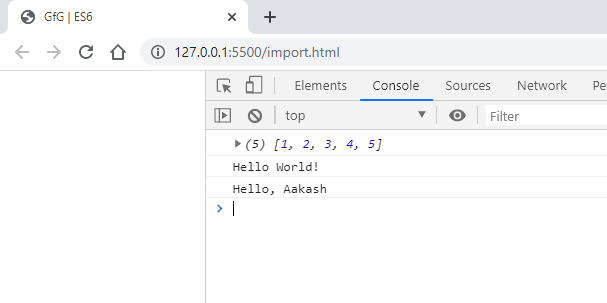Related Articles
ES6 | Import and Export
• Last Updated : 28 Feb, 2020

The ES6 is a JavaScript standard. With the help of ES6, we can create modules in JavaScript. In a module, there can be classes, functions, variables, and objects as well. To make all these available in another file, we can use export and import. The export and import are the keywords used for exporting and importing one or more members in a module.

Export: You can export a variable using the export keyword in front of that variable declaration. You can also export a function and a class by doing the same.

• Syntax for variable:
`export let variable_name;`
• Syntax for function:
```export function function_name() {
// Statements
}
```
• Syntax for class:
```export class Class_Name {
constructor() {
// Statements
}
}
```
• Example 1: Create a file named export.js and write the below code in that file.
 `export let num_set = [1, 2, 3, 4, 5];`` ` `export ``default` `function` `hello() {``    ``console.log(``"Hello World!"``);``}`` ` `export class Greeting {``    ``constructor(name) {``        ``this``.greeting = ``"Hello, "` `+ name;``    ``}``}`
• Example 2: In this example, we export by specifying the members of the module at the end of the file. We can also use alias while exporting using the as keyword.
 `let num_set = [1, 2, 3, 4, 5];`` ` `export ``default` `function` `hello() {``    ``console.log(``"Hello World!"``);``}`` ` `class Greeting {``    ``constructor(name) {``        ``this``.greeting = ``"Hello, "` `+ name;``    ``}``}`` ` `export { num_set, Greeting as Greet };`

Note: A default export should be specified here.

Import: You can import a variable using import keyword. You can specify one of all the members that you want to import from a JavaScript file.

• Syntax:
`import member_to_import from “path_to_js_file”;`
```// You can also use an alias while importing a member.
import Greeting as Greet from "./export.js";```
```// If you want to import all the members but don’t
// want to Specify them all then you can do that using
// a ' * ' star symbol.
import * as exp from "./export.js";```
• Example 1: Create a file named import.html and write the below code in that file.
 ```<``html` `lang``=``"en"``>``    ``<``head``>``        ``<``title``>Import in ES6``    ````    ``<``body``>``        ``<``script` `type``=``"module"``>`` ` `            ``// Default member first``            ``import hello, { num_set, Greeting } from "./export.js";``            ``console.log(num_set);``            ``hello();``            ``let g = new Greeting("Aakash");``            ``console.log(g.greeting);``        ````    `````
• Example 2:
 ```<``html` `lang``=``"en"``>``    ``<``head``>``        ``<``title``>Import in ES6``    ````    ``<``body``>``        ``<``script` `type``=``"module"``>``            ``import * as exp from "./export.js";`` ` `            ``// Use dot notation to access members``            ``console.log(exp.num_set);``            ``exp.hello();``            ``let g = new exp.Greeting("Aakash");``            ``console.log(g.greeting);``        ````    `````
• Output: Output will be same, importing the same file.Note: The default member should be imported first and then the none default members in curly brackets.My Personal Notes arrow_drop_up